# Feature Matching using Brute Force in OpenCV

• Difficulty Level : Hard
• Last Updated : 10 Aug, 2021

In this article, we will do feature matching using Brute Force in Python by using OpenCV library.

Prerequisites: OpenCV

OpenCV is a python library which is used to solve the computer vision problems.

OpenCV is an open source Computer Vision library. So computer vision is a way of teaching intelligence to machine and making them see things just like humans.

In other words, OpenCV is what that allows the computer to see and process visual data just like humans.

Installation:

For installing the openCV library, write the following command in your command prompt.

`pip install opencv-python`

### Approach:

• Import the OpenCV library.
• Load the images using imread() function and pass the path or name of the image as a parameter.
• Create the ORB detector for detecting the features of the images.
• Using the ORB detector find the keypoints and descriptors for both of the images.
• Now after detecting the features of the images. Now write the Brute Force Matcher for matching the features of the images and stored it in the variable named as “brute_force“.
• For matching we are using the brute_force.match() and pass the descriptors of first image and descriptors of the second image as a parameter.
• After finding the matches we have to sort that matches according to the humming distance between the matches, less will be the humming distance better will be the accuracy of the matches.
• Now after sorting according to humming distance we have to draw the feature matches for that we use drawMatches() function in which pass first image and keypoints of first image, second image and keypoints of second image and the best_matches as a parameter and stored it in the variable named as “output_image“.
• Now after drawing the feature matches we have to see the matches for that we use imshow() function which comes in cv2 library and pass the window name and output_image.
• Now write the waitkey() function and write the destroyAllWindows() for destroying all the windows.

### Oriented Fast and Rotated Brief (ORB) Detector

ORB detector stands for Oriented Fast and Rotated Brief, this is free of cost algorithm, the benefit of this algorithm is that it does not require GPU it can compute on normal CPU.

ORB is basically the combination of two algorithms involved FAST and BRIEF where FAST stands for Features from Accelerated Segments Test whereas BRIEF stands for Binary Robust Independent Elementary Features.

ORB detector first uses FAST algorithm, this FAST algorithm finds the key points then applies Harries corner measure to find top N numbers of key points among them, this algorithm quickly selects the key points by comparing the distinctive regions like the intensity variations.

This algorithm works on Key point matching, Key point is distinctive regions in an image like the intensity variations.

Now the role of BRIEF algorithm comes, this algorithm takes the key points and turn into the binary descriptor/binary feature vector that contains the combination of 0s and1s only.

The key points founded by FAST algorithm and Descriptors created by BRIEF algorithm both together represent the object. BRIEF is the faster method for feature descriptor calculation and it also provides a high recognition rate until and unless there is large in-plane rotation.

### Brute Force Matcher

Brute Force Matcher is used for matching the features of the first image with another image.

It takes one descriptor of first image and matches to all the descriptors of the second image and then it goes to the second descriptor of first image and matches to all the descriptor of the second image and so on.

Example 1: Reading/Importing the images from their path using OpenCV library.

## Python

 `# importing openCV library` `import` `cv2`   `# function to read the images by taking there path` `def` `read_image(path1,path2):` `  ``# reading the images from their using imread() function` `    ``read_img1 ``=` `cv2.imread(path1)` `    ``read_img2 ``=` `cv2.imread(path2)` `    ``return` `(read_img1,read_img2)`   `# function to convert images from RGB to gray scale ` `def` `convert_to_grayscale(pic1,pic2):` `    ``gray_img1 ``=` `cv2.cvtColor(pic1,cv2.COLOR_BGR2GRAY)` `    ``gray_img2 ``=` `cv2.cvtColor(pic2,cv2.COLOR_BGR2GRAY)` `    ``return` `(gray_img1,gray_img2)`   `# main function` `if` `__name__ ``=``=` `'__main__'``:` ` ``# giving the path of both of the images` `    ``first_image_path ``=` `'C:/UsersPython(ds)/1611755129039.jpg'` `    ``second_image_path ``=` `'C:/Users/Python(ds)/1611755720390.jpg'`   `    ``# reading the image from there path by calling the function` `    ``img1, img2 ``=` `read_image(first_image_path,second_image_path)`   `    ``# converting the readed images into the gray scale images by calling the function` `    ``gray_pic1, gray_pic2 ``=` `convert_to_grayscale(img1,img2)` `    ``cv2.imshow(``'Gray scaled image 1'``,gray_pic1)` `    ``cv2.imshow(``'Gray scaled image 2'``,gray_pic2)` `    ``cv2.waitKey()` `    ``cv2.destroyAllWindows()`

Output: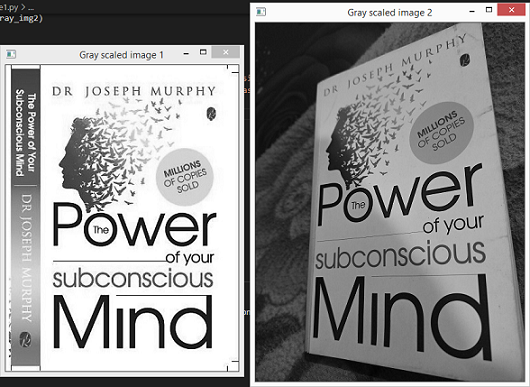Example 2: Creating ORB detector for finding the features in the images.

## Python

 `# importing openCV library` `import` `cv2`   `# function to read the images by taking there path` `def` `read_image(path1,path2):` `    ``read_img1 ``=` `cv2.imread(path1)` `    ``read_img2 ``=` `cv2.imread(path2)` `    ``return` `(read_img1,read_img2)`   `# function to convert images from RGB to gray scale ` `def` `convert_to_grayscale(pic1,pic2):` `    ``gray_img1 ``=` `cv2.cvtColor(pic1,cv2.COLOR_BGR2GRAY)` `    ``gray_img2 ``=` `cv2.cvtColor(pic2,cv2.COLOR_BGR2GRAY)` `    ``return` `(gray_img1,gray_img2)`   `# function to detect the features by finding key points and descriptors from the image` `def` `detector(image1,image2):` `    ``# creating ORB detector` `    ``detect ``=` `cv2.ORB_create()`   `    ``# finding key points and descriptors of both images using detectAndCompute() function` `    ``key_point1,descrip1 ``=` `detect.detectAndCompute(image1,``None``)` `    ``key_point2,descrip2 ``=` `detect.detectAndCompute(image2,``None``)` `    ``return` `(key_point1,descrip1,key_point2,descrip2)`   `# main function` `if` `__name__ ``=``=` `'__main__'``:` ` ``# giving the path of both of the images` `    ``first_image_path ``=` `'C:/Users/Python(ds)//1611755129039.jpg'` `    ``second_image_path ``=` `'C:/Users/Python(ds)/1611755720390.jpg'`   `    ``# reading the image from there paths` `    ``img1, img2 ``=` `read_image(first_image_path,second_image_path)`   `    ``# converting the readed images into the gray scale images` `    ``gray_pic1, gray_pic2 ``=` `convert_to_grayscale(img1,img2)`   `    ``# storing the finded key points and descriptors of both of the images` `    ``key_pt1,descrip1,key_pt2,descrip2 ``=` `detector(gray_pic1,gray_pic2)`   `    ``# showing the images with their key points finded by the detector` `    ``cv2.imshow(``"Key points of Image 1"``,cv2.drawKeypoints(gray_pic1,key_pt1,``None``))` `    ``cv2.imshow(``"Key points of Image 2"``,cv2.drawKeypoints(gray_pic2,key_pt2,``None``))`   `    ``# printing descriptors of both of the images` `    ``print``(f``'Descriptors of Image 1 {descrip1}'``)` `    ``print``(f``'Descriptors of Image 2 {descrip2}'``)` `    ``print``(``'------------------------------'``)`   `    ``# printing the Shape of the descriptors ` `    ``print``(f``'Shape of descriptor of first image {descrip1.shape}'``)` `    ``print``(f``'Shape of descriptor of second image {descrip2.shape}'``)`   `    ``cv2.waitKey()` `    ``cv2.destroyAllWindows()`

Output: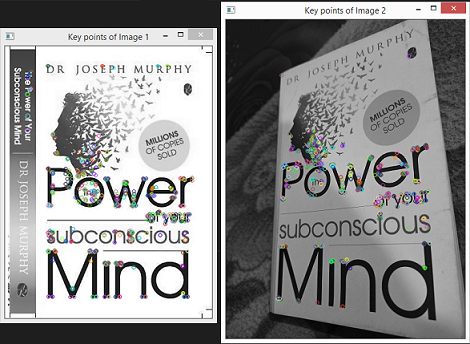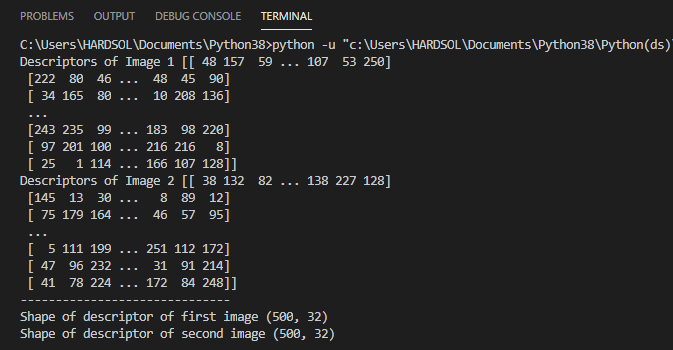The first output image shows the drawn key points of both of the images.

KeyPoints are the point of interest, in simple words means that when the human will see the image at that time the features he notices in that image, in the similar way when the machine read the image it see some points of interest known as Key points.

The second output image shows the descriptors and the shape of the descriptors.

These Descriptors are basically array or bin of numbers. These are used to describe the features, using these descriptors we can match the two different images.

In the second output image, we can see first image descriptor shape and second image descriptor shape is (467, 32) and (500,32) respectively. So, Oriented Fast and Rotated Brief (ORB) detector try to find 500 features in the image by default, and for each descriptor, it will describe 32 values.

So, now how will we use these descriptors now? We can use a Brute Force Matcher (as discussed above in the article) to match these descriptors together and find how many similarities we are getting.

Example 3: Feature Matching using Brute Force Matcher.

## Python

 `# importing openCV library` `import` `cv2`   `# function to read the images by taking there path` `def` `read_image(path1,path2):` `    ``read_img1 ``=` `cv2.imread(path1)` `    ``read_img2 ``=` `cv2.imread(path2)` `    ``return` `(read_img1,read_img2)`   `# function to convert images from RGB to gray scale ` `def` `convert_to_grayscale(pic1,pic2):` `    ``gray_img1 ``=` `cv2.cvtColor(pic1,cv2.COLOR_BGR2GRAY)` `    ``gray_img2 ``=` `cv2.cvtColor(pic2,cv2.COLOR_BGR2GRAY)` `    ``return` `(gray_img1,gray_img2)`   `# function to detect the features by finding key points ` `# and descriptors from the image` `def` `detector(image1,image2):` `    ``# creating ORB detector` `    ``detect ``=` `cv2.ORB_create()`   `    ``# finding key points and descriptors of both images using ` `    ``# detectAndCompute() function` `    ``key_point1,descrip1 ``=` `detect.detectAndCompute(image1,``None``)` `    ``key_point2,descrip2 ``=` `detect.detectAndCompute(image2,``None``)` `    ``return` `(key_point1,descrip1,key_point2,descrip2)`   `# function to find best detected features using brute force` `# matcher and match them according to there humming distance` `def` `BF_FeatureMatcher(des1,des2):` `    ``brute_force ``=` `cv2.BFMatcher(cv2.NORM_HAMMING,crossCheck``=``True``)` `    ``no_of_matches ``=` `brute_force.match(des1,des2)`   `    ``# finding the humming distance of the matches and sorting them` `    ``no_of_matches ``=` `sorted``(no_of_matches,key``=``lambda` `x:x.distance)` `    ``return` `no_of_matches`   `# function displaying the output image with the feature matching` `def` `display_output(pic1,kpt1,pic2,kpt2,best_match):`   `    ``# drawing the feature matches using drawMatches() function` `    ``output_image ``=` `cv2.drawMatches(pic1,kpt1,pic2,kpt2,best_match,``None``,flags``=``2``)` `    ``cv2.imshow(``'Output image'``,output_image)`   `# main function` `if` `__name__ ``=``=` `'__main__'``:` `    ``# giving the path of both of the images` `    ``first_image_path ``=` `'C:/Users/Python(ds)/1611755129039.jpg'` `    ``second_image_path ``=` `'C:/Users/Python(ds)/1611755720390.jpg'`   `    ``# reading the image from there paths` `    ``img1, img2 ``=` `read_image(first_image_path,second_image_path)`   `    ``# converting the readed images into the gray scale images` `    ``gray_pic1, gray_pic2 ``=` `convert_to_grayscale(img1,img2)`   `    ``# storing the finded key points and descriptors of both of the images` `    ``key_pt1,descrip1,key_pt2,descrip2 ``=` `detector(gray_pic1,gray_pic2)`   `    ``# sorting the number of best matches obtained from brute force matcher` `    ``number_of_matches ``=` `BF_FeatureMatcher(descrip1,descrip2)` `    ``tot_feature_matches ``=` `len``(number_of_matches)`   `    ``# printing total number of feature matches found` `    ``print``(f``'Total Number of Features matches found are {tot_feature_matches}'``)`   `    ``# after drawing the feature matches displaying the output image` `    ``display_output(gray_pic1,key_pt1,gray_pic2,key_pt2,number_of_matches)` `    ``cv2.waitKey()` `    ``cv2.destroyAllWindows()`

Output: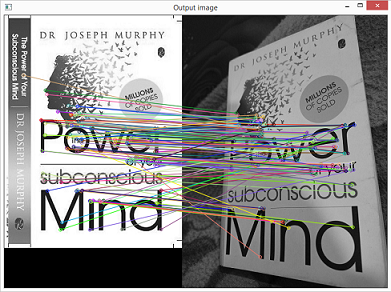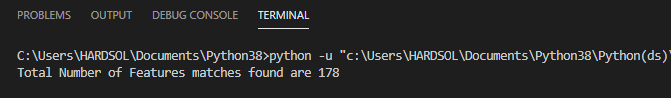We are getting total of 178 feature matches. Total 178 matches are drawn, but they are sorted according to their humming distance in ascending order means that the distance of 178th feature is greater than the first feature, so first feature match is more accurate than the 178th feature match.

It looks messy because all the 178 feature matches are drawn, let’s draw the top fifteen features (for the sake of visibility).

Example 4: First/Top fifteen Feature Matching using Brute Force Matcher.

## Python

 `# importing openCV library` `import` `cv2`   `# function to read the images by taking there path` `def` `read_image(path1,path2):` `    ``read_img1 ``=` `cv2.imread(path1)` `    ``read_img2 ``=` `cv2.imread(path2)` `    ``return` `(read_img1,read_img2)`   `# function to convert images from RGB to gray scale ` `def` `convert_to_grayscale(pic1,pic2):` `    ``gray_img1 ``=` `cv2.cvtColor(pic1,cv2.COLOR_BGR2GRAY)` `    ``gray_img2 ``=` `cv2.cvtColor(pic2,cv2.COLOR_BGR2GRAY)` `    ``return` `(gray_img1,gray_img2)`   `# function to detect the features by finding key points ` `# and descriptors from the image` `def` `detector(image1,image2):`   `    ``# creating ORB detector` `    ``detect ``=` `cv2.ORB_create()`   `    ``# finding key points and descriptors of both images ` `    ``# using detectAndCompute() function` `    ``key_point1,descrip1 ``=` `detect.detectAndCompute(image1,``None``)` `    ``key_point2,descrip2 ``=` `detect.detectAndCompute(image2,``None``)` `    ``return` `(key_point1,descrip1,key_point2,descrip2)`   `# function to find best detected features using` `# brute force matcher and match them according to there humming distance` `def` `BF_FeatureMatcher(des1,des2):` `    ``brute_force ``=` `cv2.BFMatcher(cv2.NORM_HAMMING,crossCheck``=``True``)` `    ``no_of_matches ``=` `brute_force.match(des1,des2)`   `    ``# finding the humming distance of the matches and sorting them` `    ``no_of_matches ``=` `sorted``(no_of_matches,key``=``lambda` `x:x.distance)` `    ``return` `no_of_matches`   `# function displaying the output image with the feature matching` `def` `display_output(pic1,kpt1,pic2,kpt2,best_match):` `    ``# drawing first fifteen best feature matches using drawMatches() function` `    ``output_image ``=` `cv2.drawMatches(pic1,kpt1,pic2,` `                                   ``kpt2,best_match[:``15``],``None``,flags``=``2``)` `    ``cv2.imshow(``'Output image'``,output_image)`   `# main function` `if` `__name__ ``=``=` `'__main__'``:` `    ``# giving the path of both of the images` `    ``first_image_path ``=` `'C:/Users/Python(ds)/1611755129039.jpg'` `    ``second_image_path ``=` `'C:/Users/Python(ds)/1611755720390.jpg'`   `    ``# reading the image from there paths` `    ``img1, img2 ``=` `read_image(first_image_path,second_image_path)`   `    ``# converting the readed images into the gray scale images` `    ``gray_pic1, gray_pic2 ``=` `convert_to_grayscale(img1,img2)`   `    ``# storing the finded key points and descriptors of both of the images` `    ``key_pt1,descrip1,key_pt2,descrip2 ``=` `detector(gray_pic1,gray_pic2)`   `    ``# sorting the number of best matches obtained from brute force matcher` `    ``number_of_matches ``=` `BF_FeatureMatcher(descrip1,descrip2)`   `    ``# after drawing the feature matches displaying the output image` `    ``display_output(gray_pic1,key_pt1,gray_pic2,key_pt2,number_of_matches)` `    ``cv2.waitKey()` `    ``cv2.destroyAllWindows()`

Output: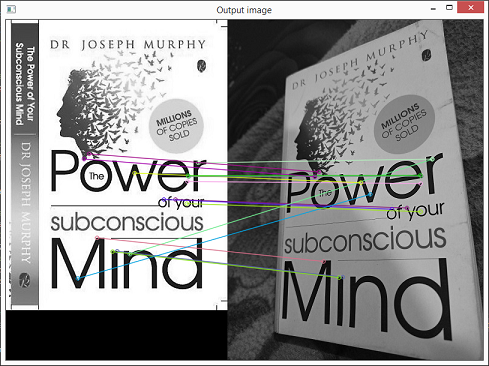The output image shows the first/top fifteen best feature matching using Brute Force Matcher.

From the above output, we can see that these matches are more accurate than all the remaining feature matches.

Let’s take another example for feature matching.

Example 5: Feature matching using Brute Force.

## Python

 `# importing openCV library` `import` `cv2`   `# function to read the images by taking there path` `def` `read_image(path1,path2):` `    ``read_img1 ``=` `cv2.imread(path1)` `    ``read_img2 ``=` `cv2.imread(path2)` `    ``return` `(read_img1,read_img2)`   `# function to convert images from RGB to gray scale ` `def` `convert_to_grayscale(pic1,pic2):` `    ``gray_img1 ``=` `cv2.cvtColor(pic1,cv2.COLOR_BGR2GRAY)` `    ``gray_img2 ``=` `cv2.cvtColor(pic2,cv2.COLOR_BGR2GRAY)` `    ``return` `(gray_img1,gray_img2)`   `# function to detect the features by finding key points and ` `# descriptors from the image` `def` `detector(image1,image2):`   `    ``# creating ORB detector` `    ``detect ``=` `cv2.ORB_create()` `    ``# finding key points and descriptors of both images` `    ``# using detectAndCompute() function` `    ``key_point1,descrip1 ``=` `detect.detectAndCompute(image1,``None``)` `    ``key_point2,descrip2 ``=` `detect.detectAndCompute(image2,``None``)` `    ``return` `(key_point1,descrip1,key_point2,descrip2)`   `# function to find best detected features using brute ` `# force matcher and match them according to there humming distance` `def` `BF_FeatureMatcher(des1,des2):` `    ``brute_force ``=` `cv2.BFMatcher(cv2.NORM_HAMMING,crossCheck``=``True``)` `    ``no_of_matches ``=` `brute_force.match(des1,des2)`   `    ``# finding the humming distance of the matches and sorting them` `    ``no_of_matches ``=` `sorted``(no_of_matches,key``=``lambda` `x:x.distance)` `    ``return` `no_of_matches`   `# function displaying the output image with the feature matching` `def` `display_output(pic1,kpt1,pic2,kpt2,best_match):` `    ``# drawing the feature matches using drawMatches() function` `    ``output_image ``=` `cv2.drawMatches(pic1,kpt1,pic2,kpt2,` `                                   ``best_match[:``30``],``None``,flags``=``2``)` `    ``cv2.imshow(``'Output image'``,output_image)`   `# main function` `if` `__name__ ``=``=` `'__main__'``:` `    ``# giving the path of both of the images` `    ``first_image_path ``=` `'C:/Users/Python(ds)/Titan_1.jpg'` `    ``second_image_path ``=` `'C:/Users/Python(ds)/Titan_nor.jpg'`   `    ``# reading the image from there paths` `    ``img1, img2 ``=` `read_image(first_image_path,second_image_path)`   `    ``# converting the readed images into the gray scale images` `    ``gray_pic1, gray_pic2 ``=` `convert_to_grayscale(img1,img2)`   `    ``# storing the finded key points and descriptors of both of the images` `    ``key_pt1,descrip1,key_pt2,descrip2 ``=` `detector(gray_pic1,gray_pic2)`   `    ``# sorting the number of best matches obtained from brute force matcher` `    ``number_of_matches ``=` `BF_FeatureMatcher(descrip1,descrip2)` `    ``tot_feature_matches ``=` `len``(number_of_matches)` `    ``print``(f``'Total Number of Features matches found are {tot_feature_matches}'``)`   `    ``# after drawing the feature matches displaying the output image` `    ``display_output(gray_pic1,key_pt1,gray_pic2,key_pt2,number_of_matches)` `    ``cv2.waitKey()` `    ``cv2.destroyAllWindows()`

Output: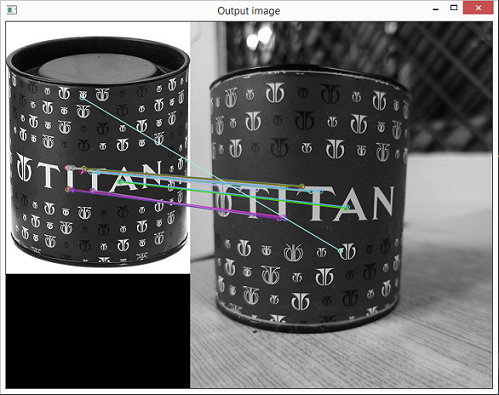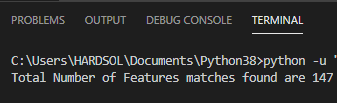In the above example we are getting total 147 best feature matches among them we are drawing only top 30 matches so that we can see the matches properly.

Example 6: Feature Matching using Brute Force Matcher by taking rotated train image.

## Python

 `# importing openCV library` `import` `cv2`   `# function to read the images by taking there path` `def` `read_image(path1,path2):` `    ``read_img1 ``=` `cv2.imread(path1)` `    ``read_img2 ``=` `cv2.imread(path2)` `    ``return` `(read_img1,read_img2)`   `# function to convert images from RGB to gray scale ` `def` `convert_to_grayscale(pic1,pic2):` `    ``gray_img1 ``=` `cv2.cvtColor(pic1,cv2.COLOR_BGR2GRAY)` `    ``gray_img2 ``=` `cv2.cvtColor(pic2,cv2.COLOR_BGR2GRAY)` `    ``return` `(gray_img1,gray_img2)`   `# function to detect the features by finding key points ` `# and descriptors from the image` `def` `detector(image1,image2):` `    ``# creating ORB detector` `    ``detect ``=` `cv2.ORB_create()`   `    ``# finding key points and descriptors of both images ` `    ``# using detectAndCompute() function` `    ``key_point1,descrip1 ``=` `detect.detectAndCompute(image1,``None``)` `    ``key_point2,descrip2 ``=` `detect.detectAndCompute(image2,``None``)` `    ``return` `(key_point1,descrip1,key_point2,descrip2)`   `# function to find best detected features using brute ` `# force matcher and match them according to there humming distance` `def` `BF_FeatureMatcher(des1,des2):` `    ``brute_force ``=` `cv2.BFMatcher(cv2.NORM_HAMMING,crossCheck``=``True``)` `    ``no_of_matches ``=` `brute_force.match(des1,des2)`   `    ``# finding the humming distance of the matches and sorting them` `    ``no_of_matches ``=` `sorted``(no_of_matches,key``=``lambda` `x:x.distance)` `    ``return` `no_of_matches`   `# function displaying the output image with the feature matching` `def` `display_output(pic1,kpt1,pic2,kpt2,best_match):` `    ``# drawing the feature matches using drawMatches() function` `    ``output_image ``=` `cv2.drawMatches(pic1,kpt1,pic2,` `                                   ``kpt2,best_match[:``30``],``None``,flags``=``2``)` `    ``cv2.imshow(``'Output image'``,output_image)`   `# main function` `if` `__name__ ``=``=` `'__main__'``:` `    ``# giving the path of both of the images` `    ``first_image_path ``=` `'C:/Users/Python(ds)/Titan_1.jpg'` `    ``second_image_path ``=` `'C:/Users/Python(ds)/Titan_rotated.jpg'`   `    ``# reading the image from there paths` `    ``img1, img2 ``=` `read_image(first_image_path,second_image_path)`   `    ``# converting the readed images into the gray scale images` `    ``gray_pic1, gray_pic2 ``=` `convert_to_grayscale(img1,img2)`   `    ``# storing the finded key points and descriptors of both of the images` `    ``key_pt1,descrip1,key_pt2,descrip2 ``=` `detector(gray_pic1,gray_pic2)`   `    ``# sorting the number of best matches obtained from brute force matcher` `    ``number_of_matches ``=` `BF_FeatureMatcher(descrip1,descrip2)` `    ``tot_feature_matches ``=` `len``(number_of_matches)` `    ``print``(f``'Total Number of Features matches found are {tot_feature_matches}'``)`   `    ``# after drawing the feature matches displaying the output image` `    ``display_output(gray_pic1,key_pt1,gray_pic2,key_pt2,number_of_matches)` `    ``cv2.waitKey()` `    ``cv2.destroyAllWindows()`

Output: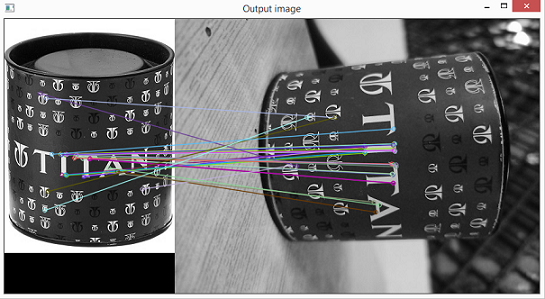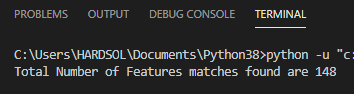In this example when we have taken the rotated train image then we have found that there is little difference in the total number of best feature matches i.e, 148.

In the first output image, we have only drawn the top thirty best feature matches.

My Personal Notes arrow_drop_up
Recommended Articles
Page :